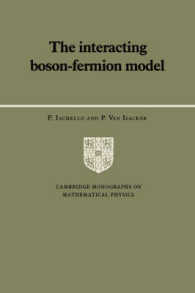### The Interacting Boson-Fermion Model (Cambridge Monographs on Mathematical Physics)

• 提携先の海外書籍取次会社に在庫がございます。通常2週間で発送いたします。
重要ご説明事項
1. 納期遅延や、ご入手不能となる場合が若干ございます。
2. 複数冊ご注文の場合、分割発送となる場合がございます。
3. 美品のご指定は承りかねます。
• ≪洋書のご注文につきまして≫ 「海外取次在庫あり」および「国内仕入れ先からお取り寄せいたします」表示の商品でも、納期の目安期間内にお届けできないことがございます。あらかじめご了承ください。

• 製本 Hardcover:ハードカバー版／ページ数 350 p.
• 言語 ENG
• 商品コード 9780521380928
• DDC分類 539.721

### 基本説明

New in paperback. Hardcover was published in 1991. Describes the mathematical framework on which the interacting boson-fermion model is built and presents applications to a variety of situations encountered in nuclei.

### Full Description

The interacting boson-fermion model has become in recent years the standard model for the description of atomic nuclei with an odd number of protons and/or neutrons. This book describes the mathematical framework on which the interacting boson-fermion model is built and presents applications to a variety of situations encountered in nuclei. The book addresses both the analytical and the numerical aspects of the problem. The analytical aspect requires the introduction of rather complex group theoretic methods, including the use of graded (or super) Lie algebras. The first (and so far only) example of supersymmetry occurring in nature is also discussed. The book is the first comprehensive treatment of the subject and will appeal to both theoretical and experimental physicists. The large number of explicit formulas for level energies, electromagnetic transition rates and intensities of transfer reactions presented in the book provide a simple but detailed way to analyse experimental data. This book can also be used as a textbook for advanced graduate students.

### Table of Contents

`Part I. The Interacting Boson-Fermion Model - 11. Operators2. Algebras3. Bose-Fermi symmetries4. Superalgebras5. Numerical studies6. GeometryPart II. The Interacting Boson-Fermion Model - 27. Operators8. Algebras9. Superalgebras10. Numerical studiesPart III. The Interacting Boson-Fermion Model-K11. The interacting boson-fermion models 3 and 4Part IV. High-Lying Collective Modes.`